Courses

# Atomic number, Isotopes and Isobars Class 11 Notes | EduRev

## Class 11 : Atomic number, Isotopes and Isobars Class 11 Notes | EduRev

The document Atomic number, Isotopes and Isobars Class 11 Notes | EduRev is a part of the Class 11 Course Chemistry for JEE.
All you need of Class 11 at this link: Class 11

Atomic Number (Z)

• The total number of protons in the nucleus of an atom gives us the atomic number of that atom.
• It is represented with the letter ‘Z.’
• All the atoms of a particular element have the same number of protons, and hence the same atomic number.
• Atoms of different elements have different atomic numbers.
• For example, all carbon atoms have the atomic number of 6, whereas all atoms of Oxygen have 8 protons in their nucleus.

Mass Number (A)

• The number of protons and neutrons combined to give us the mass number of an atom.
• It is represented using the letter ‘A.’
• As both protons and neutrons are present in the nucleus of an atom, they are together called nucleons.
• For example, an atom of carbon has 6 protons and 6 neutrons. Thus, its mass number is 12.
• While the number of protons remains the same in all atoms of an element, the number of neutrons can vary. Thus, atoms of the same element can have different mass numbers, and these are called isotopes.
• The weight of an electron is almost negligible. Thus, the atomic mass of an atom is almost the same as its mass number.

Representation of an Atom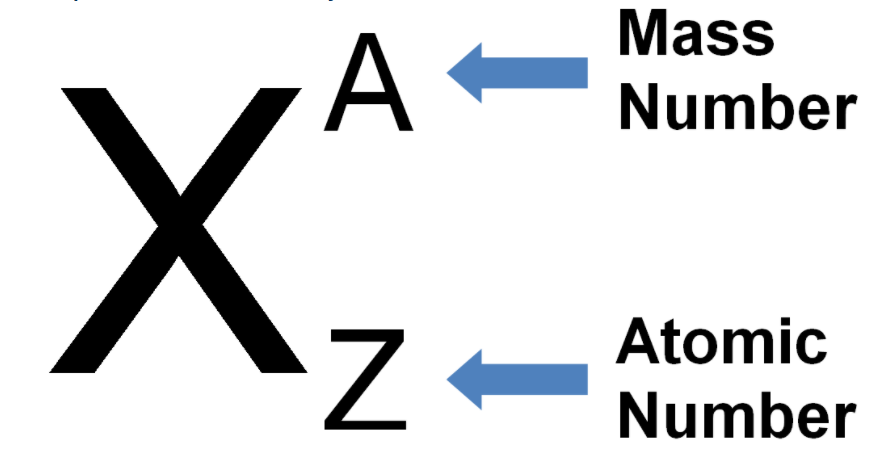Example: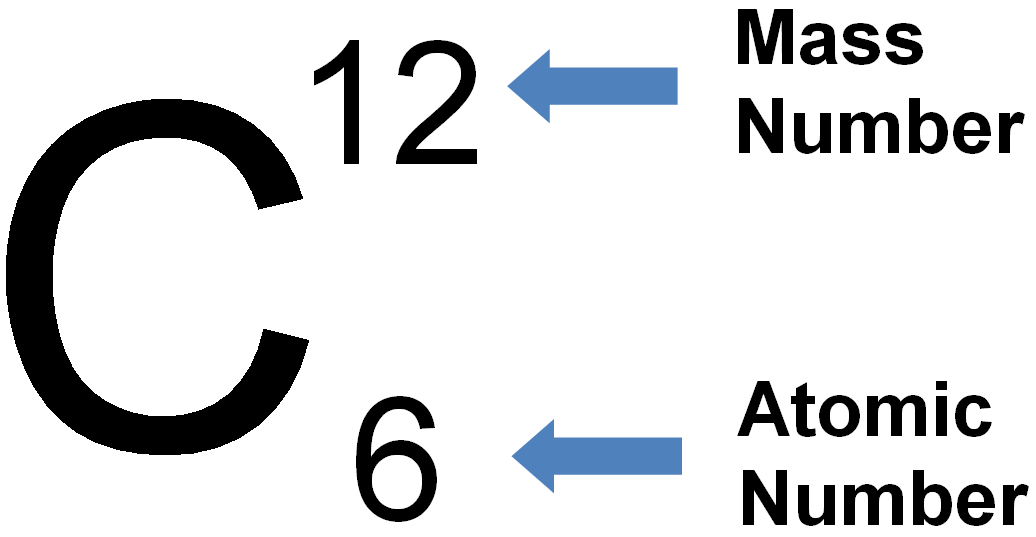Difference Between Atomic Number and Mass Number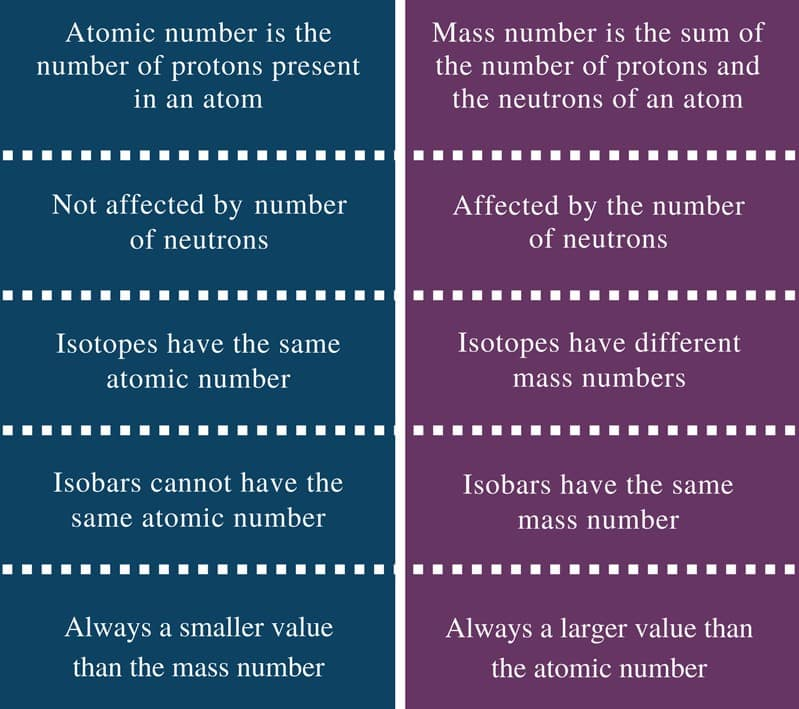Different Types of Atomic Species

(a) Isotopes

Species with the same atomic number but a different mass number are called isotopes, e.g. 1H1, 1H2, 1H3.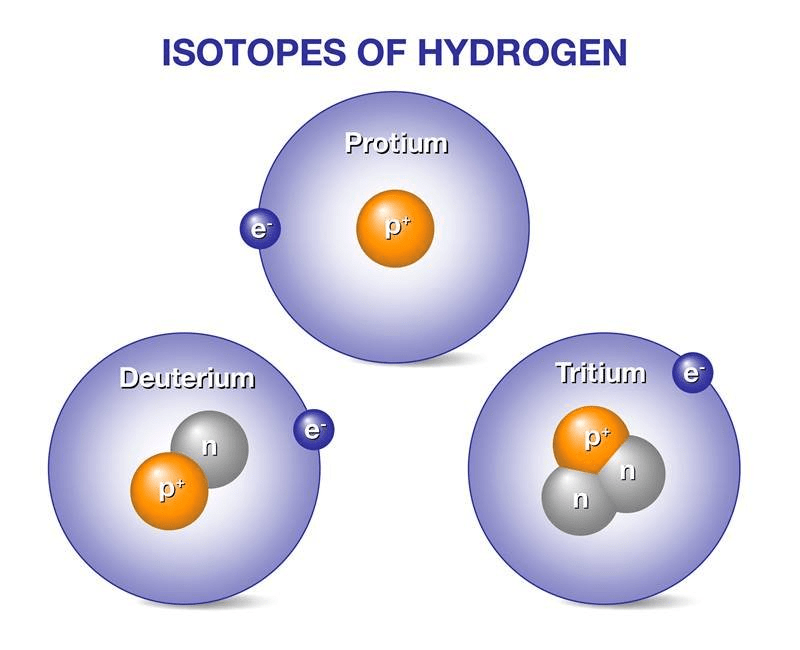Uses of Isotopes:

• It is used as uranium for the nuclear reactor
• It is used as cobalt for treating blood cancer.
• Some isotopes are used in the process of labelling for various studies. Like, isotopes of hydrogen, carbon, nitrogen etc. are used for the labelling process.
• Used for nuclear imaging of various tissues for detection of diseases. fluorine-18, gallium-67, etc. are used for this purpose.

(b) Isobars

Species with the same mass number but a different atomic number are called isobars, e.g. 18Ar4019K40, 20Ca40 all of them contain the same mass number 40 but different atomic number i.e 18, 19, and 20.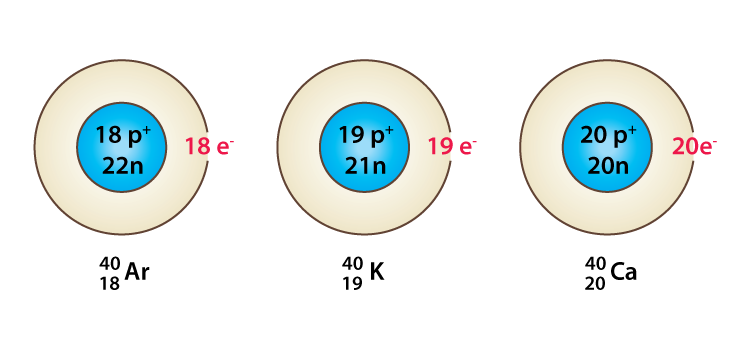Example of Isobars

Difference between Isotopes and Isobars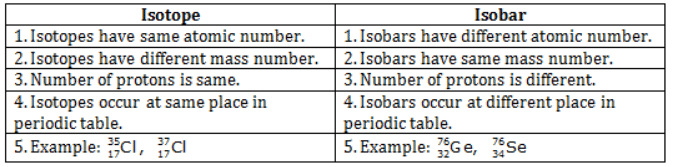(c) Isotones

Species having the same number of neutrons are called isotones, e.g. 1H3 and 2He4 are isotones.
(d) Isodiaphers

Species with the same isotopic number are called isodiaphers, e.g. 19K39, 19F9.
Isotopic number = mass number − × [2 atomic number]
(e) Isoelectronic

Species with the same number of electrons are called isoelectronic species, e.g. Na+, Mg2+.
Solved Questions

Q1: Isobars have the same number of ______?
(A) Protons
(B) Electrons
(C) Neutrons
(D) Nucleons
Solution: The answer is option 4 (Nucleons). The number of protons and neutrons alone will vary in isobars but the number of nucleons or the sum of protons and neutrons will always be the same.

Q2: Which of the following statements about Isobars is false?
(A) Their atomic numbers vary from each other.
(B) Chemically they are the same element but their forms are different.
(C) Physical properties can be similar to each other.
(D) They are different chemical elements having the same atomic mass.
Solution: Statement 2 is false. Isobars are different elements altogether.

Q3: If the atomic number of sodium is 11. Find out how many electrons and protons are present in a sodium atom.
Solution:
We know that “Atomic Number = No. of Protons = No. of Electrons”
Thus, number of electrons=11 and number of protons =11

Q4: What is the atomic number of chlorine?
(A) 18
(B) 19
(C) 17
(D) 16
Solution: The correct answer is “C”. The atomic number of chlorine is 17 because the number of protons in a chlorine atom is 17.

Q5: Isotopes of a single element vary in the number of
(A) Neutrons
(B) Protons
(C) Electrons
(D) All of the above
Solution: The answer is "A" (Neutrons).
The number of protons will always constant in a single element. However, the number of neutrons can change. The number of neutrons varies in isotopes of an element but the number of protons always remains the same.

Offer running on EduRev: Apply code STAYHOME200 to get INR 200 off on our premium plan EduRev Infinity!

## Chemistry for JEE

198 videos|450 docs|370 tests

,

,

,

,

,

,

,

,

,

,

,

,

,

,

,

,

,

,

,

,

,

,

,

,

;